## A mixture of 0.224 g of H2, 1.06 g of N2, and 0.834 g of Ar is stored in a closed container at STP. Find the volume (in L) of the container,

Question

A mixture of 0.224 g of H2, 1.06 g of N2, and 0.834 g of Ar is stored in a closed container at STP. Find the volume (in L) of the container, assuming that the gases exhibit ideal behavior.

in progress 0
6 months 2021-07-28T03:03:18+00:00 1 Answers 10 views 0

1. Answer: The volume of given container is 3.83 L.

Explanation:

Given: Mass of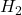= 0.224 g

Mass of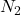= 1.06 g

Mass of Ar = 0.834 g

Since, moles is the mass of a substance divided by its molar mass. Therefore, moles of given substances present in the mixture are as follows.

Moles ofare: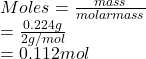Moles ofare: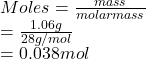Moles of Ar are: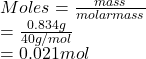Total moles = (0.112 + 0.038 + 0.021) mol = 0.171 mol

Now, using ideal gas equation the volume is calculated as follows.

PV = nRT

where,

P = pressure

V = volume

n = no. of moles

R = gas constant = 0.0821 L atm/mol K

T = temperature

Substitute the values into above formula as follows.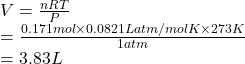Thus, we can conclude that the volume of given container is 3.83 L.